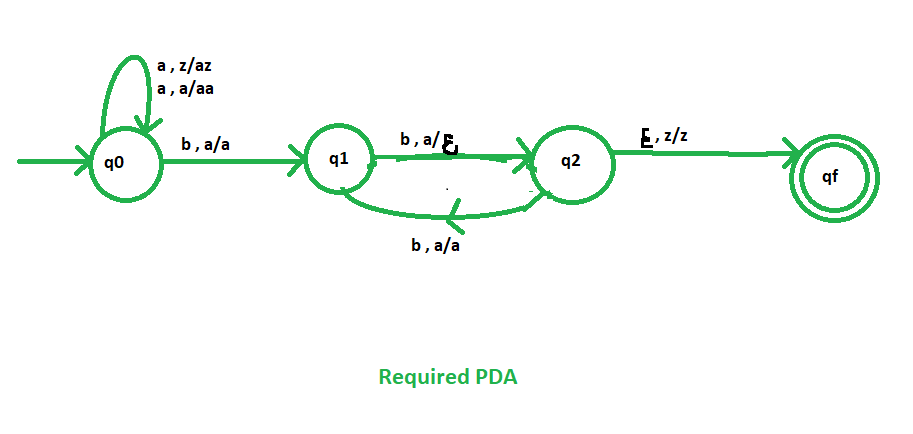# NPDA for accepting the language L = {am b(2m) | m>=1}

Prerequisite – Pushdown automata, Pushdown automata acceptance by final state

Problem – Design a non deterministic PDA for accepting the language L = {[Tex]b^{2m}   [/Tex]: m>=1}, i.e.,

L = {abb, aabbbb, aaabbbbbb, aaaabbbbbbbb, ......}

In each of the string, the number of a’s are followed by double number of b’s.

Explanation – Here, we need to maintain the order of a’s and b’s.That is, all the a’s are coming first and then all the b’s are coming. Thus, we need a stack along with the state diagram. The count of a’s and b’s is maintained by the stack.Here, the number of b’s are exactly double of the number of a’s. We will take 2 stack alphabets:= { a, z }

Where,= set of all the stack alphabet z = stack start symbol

Approach used in the construction of PDA – As we want to design a NPDA, thus every time ‘a’ comes before ‘b’. When ‘a’ comes then push it in stack and if again ‘a’ comes then also push it. After that, when ‘b’ comes then pop one ‘a’ from the stack. But we do this popping operation for alternate position of b’s, i.e., for two b’s we pop one ‘a’ and for four b’s we pop two ‘a’. So, at the end if the stack becomes empty then we can say that the string is accepted by the PDA.

Stack transition functions –(q0, a, z)(q0, az)(q0, a, a)(q0, aa)[ Indicates no operation only state change ](q0, b, a)(q1, a) [ Indicates pop operation for alternate 'b'](q1, b, a)(q2,) [ Indicates no operation only state change ](q2, b, a)(q1, a) [ Indicates pop operation for alternate 'b'](q1, b, a)(q2,)(q2,, z)(qf, z)

Where, q0 = Initial state qf = Final state= indicates pop operationSo, this is our required non deterministic PDA for accepting the language L = {[Tex]b^{2m}   [/Tex]: m>=1}.